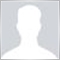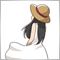Need help coding the Average of MA difference, can't get it to work properly21

So I want to code the Moving Average of TDI's MA difference and I'm at my wit's end right now. This is my code

//----- Global Variables

double   gdaRSI[]       ;
double   gdaRSI_Green[] ;
double   gdaRSI_Red[]   ;

double   TDI_MA[]       ;
double   RSI_MA_Diff[]  ;
double   MA_of_MA_Diff[];

double   BB_Top[]       ;
double   BB_Mid[]       ;
double   BB_Bot[]       ;

//+------------------------------------------------------------------+
//| Custom indicator initialization function                         |
//+------------------------------------------------------------------+

int   init()
{
//--- indicator buffers mapping

SetIndexBuffer (0, BB_Top)       ;
SetIndexStyle  (0, DRAW_LINE)    ;
SetIndexDrawBegin(0,BB_Period)   ;
SetIndexLabel  (0,"BB_Top")      ;

SetIndexBuffer (1, BB_Mid)       ;
SetIndexStyle  (1, DRAW_LINE)    ;
SetIndexDrawBegin(1,BB_Period)   ;
SetIndexLabel  (1,"BB_Mid")      ;

SetIndexBuffer (2, BB_Bot)       ;
SetIndexStyle  (2, DRAW_LINE)    ;
SetIndexDrawBegin(2,BB_Period)   ;
SetIndexLabel  (2,"BB_Bot")      ;

SetIndexBuffer (3, gdaRSI_Green) ;
SetIndexStyle  (3, DRAW_LINE)    ;
SetIndexDrawBegin(3,RSI_Period)  ;
SetIndexLabel  (3,"TDI_Main")    ;

SetIndexBuffer (4, gdaRSI_Red)   ;
SetIndexStyle  (4, DRAW_LINE)    ;
SetIndexDrawBegin(4,RSI_Period)  ;
SetIndexLabel  (4,"TDI_Signal")  ;

SetIndexBuffer (5, TDI_MA)       ;
SetIndexStyle  (5, DRAW_LINE)    ;
SetIndexDrawBegin(5,RSI_Period)  ;
SetIndexLabel  (5,"TDI_MA")      ;

SetIndexBuffer (6, gdaRSI)       ;
SetIndexStyle  (6, DRAW_NONE)    ;
SetIndexDrawBegin(6,RSI_Period)  ;
SetIndexLabel  (6,"RSI")         ;

IndicatorDigits(3);

//---
return(0);
}

//+------------------------------------------------------------------+
//| Custom Indicator On-Tick Calculation                             |
//+------------------------------------------------------------------+

int   start()
{

int   iNewBars, iCountedBars, i;

//-- Get unprocessed bars

iCountedBars    =  IndicatorCounted()   ;

if( iCountedBars < 0 ) return (-1)      ;

if( iCountedBars > 0 ) iCountedBars--   ;

iNewBars  =  MathMin(Bars-iCountedBars, Bars-1);

//-----> Calculate TDI and Bollinger Bands

for( i = iNewBars - 1; i >= 0; i-- )
{
gdaRSI[i]         =  iRSI(NULL,0,RSI_Period,RSI_Price,i);
}

for( i = iNewBars - 1; i >= 0; i-- )
{
gdaRSI_Green[i]   =  iMAOnArray(gdaRSI,0,RSI_Green_Period,0,RSI_Green_Mode,i);
gdaRSI_Red[i]     =  iMAOnArray(gdaRSI,0,RSI_Red_Period,0,RSI_Red_Mode,i);
BB_Mid[i]         =  iMAOnArray(gdaRSI,0,BB_Period,0,BB_Mode,i);
BB_Top[i]         =  BB_Mid[i] + BB_StdDev * iStdDevOnArray(gdaRSI,0,BB_Period,0,BB_Mode,i);
BB_Bot[i]         =  BB_Mid[i] - BB_StdDev * iStdDevOnArray(gdaRSI,0,BB_Period,0,BB_Mode,i);
}

for( i = iNewBars - 1; i >= 0; i-- )      RSI_MA_Diff[i]    =  gdaRSI_Green[i] - gdaRSI_Red[i];

for( i = iNewBars - 1; i >= 0; i-- )      MA_of_MA_Diff[i]  = iMAOnArray(RSI_MA_Diff,0,TDI_MA_Period,0,TDI_MA_Mode,i);

for( i = iNewBars - 1; i >= 0; i-- )      TDI_MA[i]         = gdaRSI_Red[i] + MA_of_MA_Diff[i];	//********** THIS LINE **********//

Print("BB_Bot-       = ",BB_Bot," ===> ",BB_Bot);
Print("BB_Mid-       = ",BB_Mid," ===> ",BB_Mid);
Print("BB_Top-       = ",BB_Top," ===> ",BB_Top);
Print("TDI_MA-       = ",TDI_MA," ===> ",TDI_MA);
Print("gdaRSI_Red-   = ",gdaRSI_Red," ===> ",gdaRSI_Red);
Print("gdaRSI_Green- = ",gdaRSI_Green," ===> ",gdaRSI_Green);
Print("gdaRSI-       = ",gdaRSI," ===> ",gdaRSI);
Print(" ||----- Indicator Value -----|| " );

IndicatorShortName("TDI-MA v1 by iJoe");

return(0);

}

All other buffer is working fine and is showing in the MT4 seperated window except for the TDI_MA[i]

Normally I would print indicator value out to check if there is any error in the calculation but I don't know why the Print function doesn't print anything for me when I test it in MT4475

Buffers are not allocated for RSI_MA_Diff and MA_of_MA_Diff.

SetIndexBuffer (6 , gdaRSI)       ;
SetIndexBuffer (7 , RSI_MA_Diff)  ;
SetIndexBuffer (8 , MA_of_MA_Diff);

//SetIndexStyle  (6, DRAW_NONE)    ;
//SetIndexDrawBegin(6,RSI_Period)  ;
//SetIndexLabel  (6,"RSI")         ;21

It worked! Thank you @Naguisa Unada